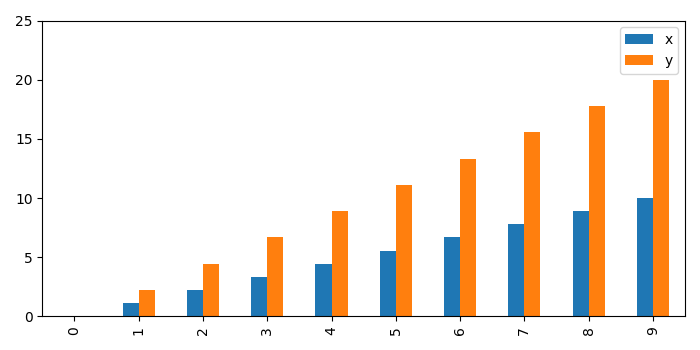# Setting Y-axis in Matplotlib using Pandas

To set Y-Axis in matplotlib using Pandas, we can take the following steps −

• Create a dictionary with the keys, and y.

• Create a data frame using Pandas.

• Plot data points using Pandas plot, with ylim(0, 25) and xlim(0, 15).

• To display the figure, use show() method.

## Example

import numpy as np
import pandas as pd
from matplotlib import pyplot as plt
plt.rcParams["figure.figsize"] = [7.00, 3.50]
plt.rcParams["figure.autolayout"] = True
d = dict(
x=np.linspace(0, 10, 10),
y=np.linspace(0, 10, 10)*2
)
df = pd.DataFrame(d)
df.plot(kind="bar", ylim=(0, 25), xlim=(0, 15))
plt.show()

## Output